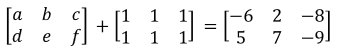At this point in our Linear Algebra course we have worked with matrices extensively, we have done row operations to solve systems of linear equations by representing a linear system as a matrix and use them to our advantage through row reduction and echelon forms. We have also learned to understand not only the notation of matrices, but their elements, their dimensions and it is time now we think of them as a whole and learn what operations can be done with them (notice "with them", NOT "in them").

On this lesson we will introduce the first two out of four basic operations that can be done with a pair (or more) matrices: addition, subtraction, scalar multiplication and multiplication. We know these basic operations from arithmetics and so we just need to learn how they work with the whole structure of the matrices and the results they produce. Notice we did not mention matrix division, that is because division among matrices does not exist (or at least the process hasn't been defined yet), the only division we can do is to divide the elements in a matrix by a scalar, but divide a matrix by another matrix is not possible.

### Addition and subtraction of matrices

We start by working through the two most basic operations we have known since we first were introduced to mathematics in elementary school: addition and subtraction (or "plus" and "minus" something). In order to work through these operations on this lesson let us define a set of two matrices to add and subtract. And so, we define matrices A and B as follows:

Now with them, we set up to work through the operations of adding and subtracting matrices. A few rules will apply to the operation, but we will talk about them in the next section, for now, let us go through the process quickly and then we can come back and check how the operations were possible. Let us start by adding A and B:

We have color coded each element in the matrices so you can see what was done in each element's case. Notice how addition of two matrices acts on a one-by-one element case, requiring that you have the exact same of elements in the matrices involved in the operation, and that they should be distributed in the same manner.

We will retake this topic on the next section, but for now, you must remember that when adding matrices you will be adding the elements of the first matrix with the corresponding one (the one in the "same spot", same row and column number) in the second matrix.

Now let us take a look into matrix subtraction:

Looking at the subtraction above we can easily conclude that the process heavily resembles that of addition since the subtraction is computed on a one-by-one case in each of the elements of the matrices, thus producing a result for each corresponding element space and resulting in a matrix with the same number of elements in the same organization.

In simple words, addition and subtraction of matrices work the same, the only difference between them is the arithmetic sign you use to operate: the plus sign for addition and the minus sign for subtraction.

It is imperative you understand this conclusion since this may help you on not falling into confussions when dealing with different literature on the topic. The thing is, some mathematicians, books, or study materials in general, may refer to the addition and subtraction operations both as "addition" in general. This particular reference comes from the fact that subtraction happens to be equivalent to the addition of numbers where at least one of them is negative. If you have any questions on this remember that subtraction in arithmetics is also called "the difference" and is all because when you add numbers with different signs the result is the difference between them. We have covered such topic in our lesson on subtracting integers, which although not part of this Linear Algebra course, you can check out any time on the StudyPug website. You can also check the next article on addition and subtraction of integers which contains extra exercises plus some graphic representations.

In summary: How to add matrices? We add each corresponding element on the involved matrices to produce a new matrix where such elements will occupy the same spot as their predecessors. How to subtract matrices? In exactly the same manner as we compute addition, except that we use a minus sign to operate instead of a plus sign.

### Rules for adding and subtracting matrices

Matrix addition and subtraction are two operations which although straightforward and simple to resolve (as shown above), you still have a few rules to follow in order to operate.

For example, when we were adding matrix A to matrix B above, notice first that A and B are very similar matrices, they happen to have the same amount of rows and columns each and the operation of addition applied to every "corresponding term" in them. This brings about the main rule of adding and subtracting matrices which corresponds to the dimensions of the matrices that are being operated on with one another.

Also notice that subtracting matrices follows a very similar regime, in reality the only change is that instead of having an addition sign in between the matrices and the corresponding elements in each matrix space, you have a subtraction sign.

With all of this in mind, the rules for adding and subtracting matrices are:

1. You can only add or subtract matrices which have the exact same dimensions.
1. If you think about it, this comes from the fact that adding matrices with different dimensions creates an issue because not all the elements in each matrix will have a corresponding element to operate with, and so, making the operation impossible to complete.
2. Addition of matrices is commutative, but subtraction is not unless you apply the negative sign to the matrix on the right first thus converting the operation into an addition first.
1. The commutative property means that while adding two matrices, you can change the order in which you add them and this will not affect the result.
2. This is not as simple when subtracting. In equation 3 we have an example of a matrix subtraction, notice that if you just switch the order of the matrices without moving the negative sign the result would not be the same, then, how can subtraction of matrices be commutative too? You have to apply the negative sign to the matrix that is being subtracted first! Check the process step by step below:
Notice how in order to be able to change the order of the matrices without affecting the result, we have to convert the subtraction into an addition, which just means that you have to apply the negative sign to the second matrix first, thus making all of its elements negative numbers (which will produce the subtraction operation without worrying about the order of the elements). This is the only way how you can commute the order of matrices while subtracting, so remember this process when working on even more complicated problems: such as cases in which you may have more than two matrices and are associating within groups. More on association in our next point.
3. Matrix addition and subtraction are associative.
1. The associative property is used in problems involving more than two matrices which are being added or subtracted from one another. This property allows you to work on the operation between two of the matrices first, and then use the matrix obtained from such operation to work through the second operation at hand. Repeat such process until you have gone through all of the needed operations involving all of the matrices in the problem.
4. When adding or subtracting matrices, the resulting matrix will have the same dimensions as the matrix that produced it.
1. This makes sense since each element on the first matrix will operate on the corresponding element of the second matrix, thus producing a result for each element space. Such results will then become the elements of the new matrix.

Next we are about to show examples of addition and subtraction of matrices. In the first example exercise we will focus on showing the conditions in which such operations are possible. Then we solve a few examples of matrix problems with the operations explained step by step. We end this section by solving matrix equations which involve either addition or subtraction of matrices.

Example 1

Can we add or subtract the following matrices?

• Case 1:
Notice the dimensions of the matrices involved in the addition are not the same. The first matrix has three rows and two columns, thus this is a 3x2 matrix, while the second one contains two rows and three columns, making its dimensions equal to 2x3. Having such differences, this means that although they have the same amount of elements each, their positions do not correspond with one another, and so by matrix addition rules, the addition is not possible.
• Case 2:
Given that in this case we would be adding 2x2 matrices, (explicitly; both matrices have the same dimension of 2x2) then yes, the addition of these two matrices is possible by adding each of the elements in the first matrix with its corresponding one from the second matrix. The resulting matrix will also have as dimensions 2x2.
• Case 3:
As you may be already thinking, adding 2 matrices such as the ones above is not possible. The dimensions of these matrices are not the same (one is a 3x3 matrix while the other one is a 2x2) and so, this addition is not possible.

Example 2

When adding two matrices, each element gets added to its corresponding element in the other matrix. The process is simple and is shown below:

Follow the same process in the next two exercises.

Example 3

And so, we add each element on the matrices to its corresponding one in the other matrix. Notice that, as mentioned before, the matrices must have the same dimensions in order for this element-by-element correspondence to occur:

Example 4

When adding more than two matrices, you can associate two by performing their addition first, and then add the resulting matrix to the matrix that is left. This is what we call the associative property of matrix addition, this and more properties of matrix addition will be explained in later lessons, for now, check the process for this problem below:

So as you can see, the first step was to add matrices one and two, which results in a new matrix that is later added to the third one. If a matrix addition contains more thna 3 matrices, the same association process can be repeated as many times as necessary to simplify the problem until you have a simple two-matrix operation.

Example 5

Subtract the following matrices:

Once more, notice that the matrices involved in a subtraction must have the same dimensions so the element-by-element correspondence is met.

Example 6

Subtract the following matrices:

No matter how big the matrices are, as long as they have the same dimensions you can perform the subtraction.

Example 7

Subtract the following matrices:

For a matrix subtraction with more than two matrices, notice that we can use the associative property again. Remember that matrix subtraction is not commutative (you cannot change the order of the matrices in the operation and obtain the same result).

Example 8

Solve the following equation containing an addition of a constant matrix to a variable.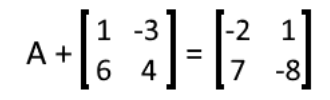Equation 20: Solve for A in the matrix equation (part 1)

We start by subtracting the matrix in the left hand side to both sides of the equation so we can solve for A easily by performing a matrix subtraction: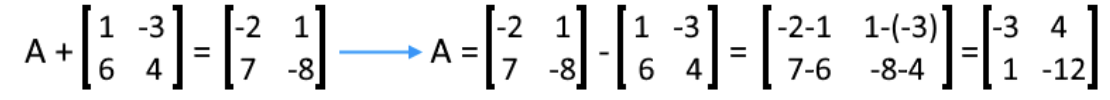Equation 21: Solve for A in the matrix equation (part 2)

Example 9

Solve the following matrix equations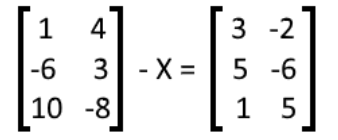Equation 22: Solve for X in the matrix equation (part 1)

On this case, the simplest way to solve for X is to pass it to the right hand side by adding it to both sides of the equation so it becomes positive. We also subtract the matrix found in the right hand side of the equation from both sides, and so, we end up with a matrix subtraction that defines X.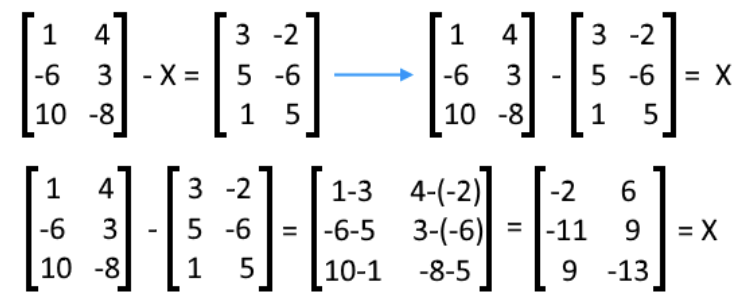Equation 23: Solve for X in the matrix equation (part 2)

By solving the matrix subtraction we find X.

Example 10

Find the values of the elements a, b, c, d, e, and f by solving the following matrix equation in the most efficient manner.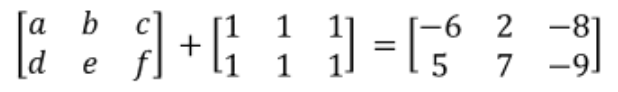Equation 24: Finding the values of the elements in the left-most matrix

On this problem we can translate element by element case, an equation that will allow us to obtain the value of each of the elements in the first matrix.

In other words, you know the element from the first row and first column (top left) in the first matrix, will be added to the element in the first row and first column on the second matrix, to obtain the element in that space in the resulting matrix on the right hand side. In other words, we can easily transcribe the next equations for each element space:

And from that, we can solve the value of each variable:

After learning how to add and subtract matrices, it is time for us to look into other operations: scalar multiplication of matrices and multiplication of two or more matrices will be explained in the next two lessons.

We finalize our lesson for today with a few useful links: the first one contains a quick list of the basic rules of matrix addition and an extra lecture on matrix operations.

And this is it, see you in our next lesson!
##### Don't just watch, practice makes perfect

In this lesson, we will learn how to add and subtract matrices. We can only add or subtract matrices if their dimensions are the same. To add matrices, we simply add the corresponding matrix elements together. To subtract matrices, we simply subtract the corresponding matrix elements together. Not only can we add and subtract matrices, but we can solve matrix equations as well. For example, we can add or subtract both sides of the matrix equations to find out what the variable is.
Basic Concepts: Notation of matrices

#### Lessons

We can add and subtract matrices only if the dimensions of the matrices are the same. For example,

Let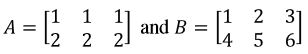We want to find out what A+B is. We see that we are allowed to add A and B together because both A and B has a dimension of 2 x 3.

To add the two matrices together, we simply just need to add the corresponding matrix elements of A and B. So,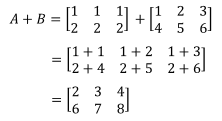Same idea goes for subtracting. We can subtract them because the dimensions are the same. If we want to find out what A - B is, then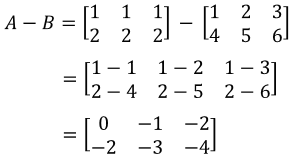• Introduction

• 1.
Understanding how to add and subtract matrices
Can we add or subtract the following matrices?
a)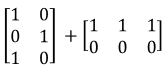b)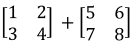c)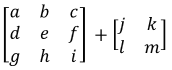• 2.
a)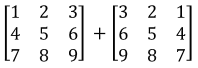b)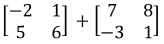c)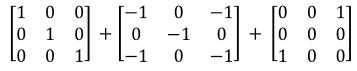• 3.
Subtracting matrices
Subtract the following matrices
a)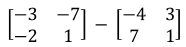b)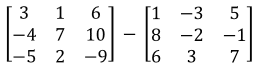c)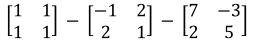• 4.
Solving matrix equations
Solve the following matrix equations:
a)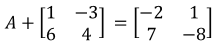b)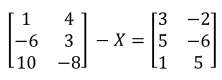c)